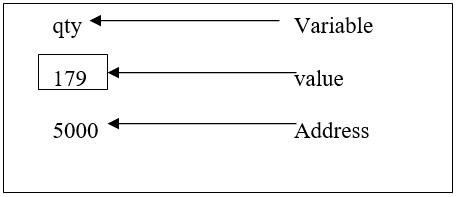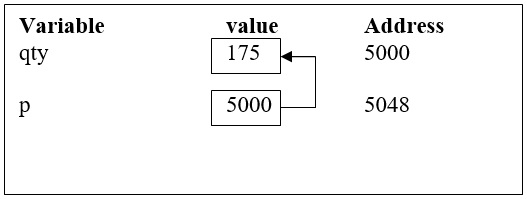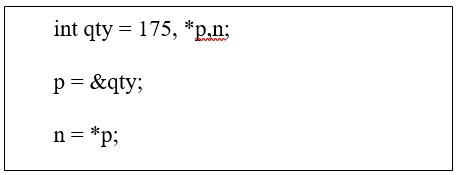# What are different pointer operations and problems with pointers in C language?

CServer Side ProgrammingProgramming

A pointer is a variable whose value is the address of an another variable, i.e., direct address of the memory location. Like any variable or constant, you must declare a pointer before using it to store any variable address.

Consider the following statement −

int qty = 179;

The representation of the variable in memory is as follows −You can declare a pointer as follows −

Int *p;

It means ‘p’ is a pointer variable that holds the address of another integer variable.

Address operator (&) is used to initialize a pointer variable.

For Example −

int qty = 175;
int *p;
p= &qty;To access the value of the variable, indirection operator (*) is used.

For example −‘*’ can be treated as value at address.

The two statements are equivalent to the following statement −

 p = &qty;
n = *p; n =qty

## Different pointer operations

The pointer operations in C language are explained below −

• Assignment − We can assign an address to a pointer by using & (address operator).

• Value finding − It is nothing but dereferencing, where the * operator gives the value stored in the pointed to location.

• Taking a pointer address − Just like other variables, pointer variables have an address as well as a value, with the help of address operator we can find were the pointer itself is stored.

• Adding an integer to a pointer − We can use + operator to add an integer to a pointer or a pointer to an integer. Here, in both the cases the int is multiplied with the no of bytes in the pointed to type, and the result is added to original address.

• Incrementing a pointer − It is an array element that makes to move to the next element of the array.

• Subtracting an int from a pointer − We are using – (minus) operator to subtract an integer from a pointer. The integer is multiplied with the number of bytes in pointed to type, and the result is subtracted from original address.

• Decrementing a pointer − Decrementing pointer, points to previous location instead of before, we can use both pre and postfix form for decrement operator.

• Subtraction − We can find difference of two pointers. Generally, we used to find out how apart the elements are.

• Comparison − We will use relational operator to compare the values of two pointers.

## Example

Given below is the program to demonstrate the functioning of the pointer operations in C language −

#include<stdio.h>
main ( ){
int x,y;
//Declaring a pointer
int *p;
clrscr ( );
x= 10;
//Assigning value to a pointer
p = &x;
y= *p;
printf ("Value of x = %d", x);
printf ("x is stored at address %u", &x);
printf ("Value of x using pointer = %d", *p);
printf ("address of x using pointer = %u", p);
printf (“value of x in y = %d", y);
*p = 25;
printf ("now x = %d", x)
getch ( );
}

## Output

When you execute the above mentioned program, you get the following output −

Value of x = 10
x is stored at address = 5000
Address of x using pointer = 10
Address of x using pointer = 5000
Value of x in y = 10
Now x = 25